## Measures of Variance

### Common Measures of Variance

Range

The range is the difference between the high and low values. Since it uses only the extreme values, it is greatly affected by extreme values.

Procedure for finding

1. Take the largest value and subtract the smallest value

FormulaVariance

The variance is the average squared deviation from the mean. It usefulness is limited because the units are squared and not the same as the original data. The sample variance is denoted by s2, it is an unbiased estimator of the population variance.

Procedure for finding

1. Find the mean of the data
2. Subtract the mean from each value to find the deviation from the mean
3. Square the deviation from the mean
4. Total the squares of the deviation from the mean
5. Divide by the degrees of freedom (one less than the sample size)

Formula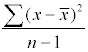Standard Deviation

The standard deviation is the average deviation from the mean. It is found by taking the square root of the variance and solves the problem of not having the same units as the original data. The sample standard deviation is denoted by s. It is not an unbiased estimator of the population standard deviation.

Procedure for finding

1. Find the variance
2. Take the square root

Formula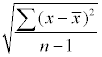### Less Common Measures of Variance

Mean Absolute Deviation

The sum of the deviations from the mean will always be zero. We need to make sure that none of the deviations are negative. We can do this by squaring each deviation (as we do in the variance or standard deviation) or by taking the absolute value (as we do in the mean absolute deviation).

Procedure for finding

1. Find the mean of the data
2. Subtract the mean from each data value to get the deviation from the mean
3. Take the absolute value of each deviation from the mean
4. Total the absolute values of the deviations from the mean
5. Divide the total by the sample size.

Formula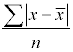Variation

The variation is the sum of the squares of the deviations from the mean. It has units that are squared instead of the same as the original data and it does not take the sample size into account.

Procedure for finding

1. Find the mean of the data
2. Subtract the mean from each value to find the deviation from the mean
3. Square the deviation from the mean
4. Total the squares of the deviation from the mean

Formula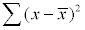Range Rule of Thumb

The range rule of thumb says that the range is approximately four times the standard deviation. Alternatively, the standard deviation is approximately one-fourth the range. That means that most of the data lies within two standard deviations of the mean.

Procedure for finding

1. Find the range
2. Divide it by four

Formula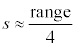Pearson's Index of Skewness

Pearson's index of skewness can be used to determine whether the data is symmetric or skewed. If the index is between -1 and 1, then the distribution is symmetric. If the index is no more than -1 then it is skewed to the left and if it is at least 1, then it is skewed to the right.

Procedure for finding

1. Find the mean, median, and standard deviation of the data.
2. Subtract the median from the mean.
3. Multiply by 3
4. Divide by the standard deviation

Formula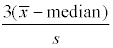Coefficient of Variation

The coefficient of variation is expressed as a percent and describes the standard deviation relative to the mean. It can be used to compare variability when the units are different (the units will divide out, providing just a raw number).

Procedure for finding

1. Find the mean and standard deviation for the data
2. Divide the standard deviation by the mean
3. Multiply by 100

Formula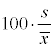Chebyshev's Rule

A rule that states the minimum amount of data that will like within k (k>1) standard deviations of the mean for any distribution of data. There will be at least 3/4 (75%) of the data within 2 standard deviations of the mean and at least 8/9 (89%) of the data within 3 standard deviations of the mean.

The rule states

At least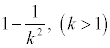of the data will like within k standard deviations of the mean

To verify the rule

1. Rank the data from lowest to highest. This is not necessary, but it makes it easier.
2. Find the mean and standard deviation of the data.
3. Find the lower boundary by multiplying the standard deviation by k and subtracting from the mean.
4. Find the upper boundary by multiplying the standard deviation by k and adding it to the mean.
5. Count the number of values between these two boundaries.
6. Divide the number of values between the boundaries by the total number of values

Empirical Rule

While Chebyshev's rule works for any distribution of data, the empirical rule only works for bell-shaped, symmetric data. It is, however, more precise than Chebyshev's rule.

The rule states

• Approximately 68% of the values will lie within one standard deviation of the mean
• Approximately 95% of the values will lie within two standard deviations of the mean
• Approximately 99.7% of the values will lie within three standard deviations of the mean

The empirical rule is sometimes called the "68-95-99.7 Rule".To verify the rule

1. Rank the data from lowest to highest. This is not necessary, but it makes it easier.
2. Find the mean and standard deviation of the data.
3. Find the lower boundary by multiplying the standard deviation by k and subtracting from the mean.
4. Find the upper boundary by multiplying the standard deviation by k and adding it to the mean.
5. Count the number of values between these two boundaries.
6. Divide the number of values between the boundaries by the total number of values